## How To Calculate p-Value in Excel

The theory behind p-values and the null hypothesis might seem complicated at first, but understanding the concepts will help you navigate the world of statistics. Unfortunately, these terms are often misused in popular science, so it would be useful for everyone to understand the basics.Calculating the p-value of a model and proving/disproving the null hypothesis is surprisingly simple with MS Excel. There are two ways to do it and we’ll cover both of them. Let’s dig in.

## Null Hypothesis and p-Value

The null hypothesis is a statement, also referred to as a default position, which claims that the relationship between the observed phenomena is non-existent. It can also be applied to associations between two observed groups. During the research, you test this hypothesis and try to disprove it.

For example, say you want to observe whether a particular fad diet has significant results. The null hypothesis, in this case, is that there is no significant difference in the test subjects’ weight before and after dieting. The alternative hypothesis is that the diet did make a difference. This is what researchers would try to prove.

The p-value represents the chance that the statistical summary would be equal to or greater than the observed value when the null hypothesis is true for a certain statistical model. Though it is often expressed as a decimal number, it is generally better to express it as a percentage. For example, the p-value of 0.1 should be represented as 10%.

A low p-value means that the evidence against the null hypothesis is strong. This further means that your data is significant. On the other hand, a high p-value means that there’s no strong evidence against the hypothesis. To prove that the fad diet works, researchers would need to find a low p-value.

A statistically significant result is the one that is highly unlikely to happen if the null hypothesis is true. The significance level is denoted with the Greek letter alpha and it has to be bigger than the p-value for the result to be statistically significant.

Many researchers in a wide range of fields use the p-value to get a better and deeper insight into the data they’re working with. Some of the prominent fields include sociology, criminal justice, psychology, finance, and economics.

## Finding the p-Value in Excel 2010

You can find the p-value of a data set in MS Excel via the T-Test function or using the Data Analysis tool. First, we’ll look into the T-Test function. We’ll examine five college students that went on a 30-day diet. We’ll compare their weight before and after the diet.

NOTE: For the purposes of this article, we’ll split it into MS Excel 2010 and 2016. Though the steps should generally apply to all versions, the layout of the menus and whatnot will differ.

### T-Test Function

Follow these steps to calculate the p-value with the T-Test function.

1. Create and populate the table. Our table looks like this:2. Click on any cell outside your table.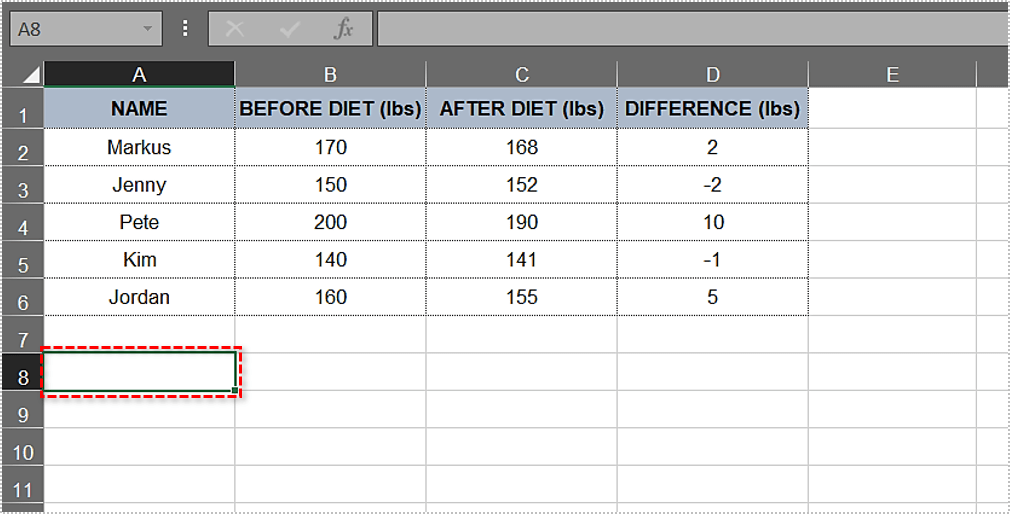3. Type in: =T.Test(.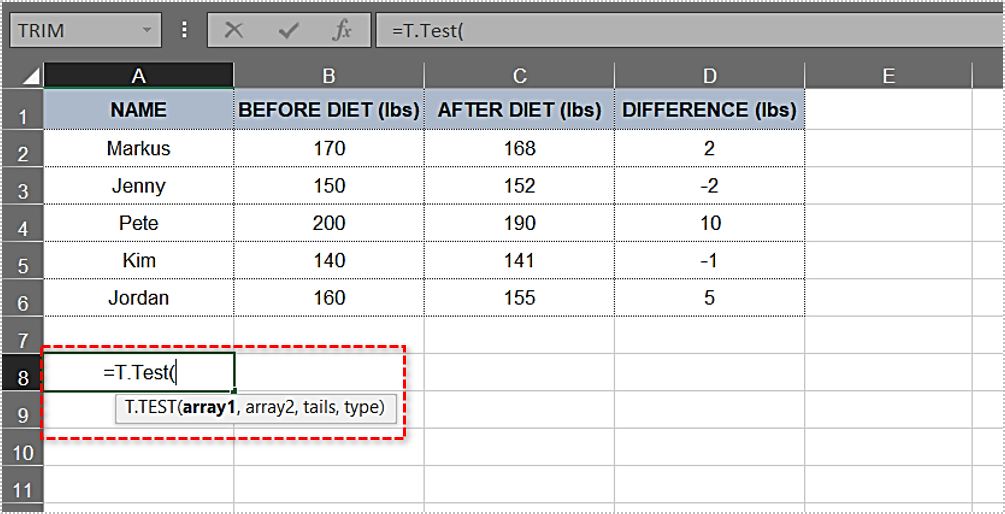4. After the open bracket, type in the first argument. In this example, it is the Before Diet column. The range should be B2:B6. Thus far, the function looks like this: T.Test(B2:B6.5. Next, we’ll enter the second argument. The After Diet column and its results are our second argument and the range we need is C2:C6. Let’s add it to the formula: T.Test(B2:B6,C2:C6.6. Type in a comma after the second argument and the one-tailed distribution and two-tailed distribution options will automatically appear in a drop-down menu. Let’s pick the first – one-tailed distribution. Double-click on it.7. Type in another comma.8. Double-click on the Paired option in the next drop-down menu.9. Now that you have all the elements you need, close the bracket. The formula for this example looks like this: =T.Test(B2:B6,C2:C6,1,1)10. Press Enter. The cell will display the p-value immediately. In our case, the value is 0.133905569 or 13.3905569%.Being higher than 5%, this p-value doesn’t provide strong evidence against the null hypothesis. In our example, the research didn’t prove that dieting helped the test subjects lose a significant amount of weight. This doesn’t necessarily mean the null hypothesis is correct, only that it hasn’t been disproven yet.

### Data Analysis Route

The Data Analysis tool lets you do many cool things, including p-value calculations. To make things simpler, we’ll use the same table as in the previous method.

Here’s how it’s done.

1. Since we already have the weight differences in the D column, we’ll skip the difference calculation. For the future tables, use this formula: =”Cell 1”-“Cell 2”.

2. Next, click on the Data tab in the Main menu.3. Select the Data Analysis tool.4. Scroll down the list and click the t-Test: Paired Two Sample for Means option.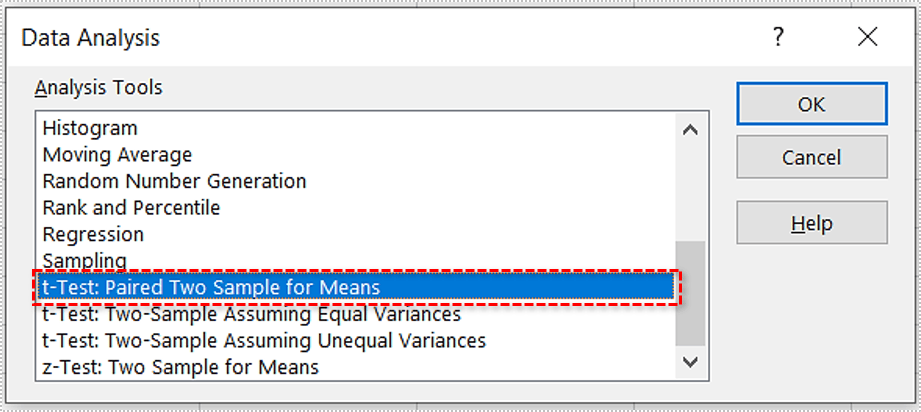5. Click OK.6. A pop-up window will appear. It looks like this:7. Enter the first range/argument. In our example, it is B2:B6.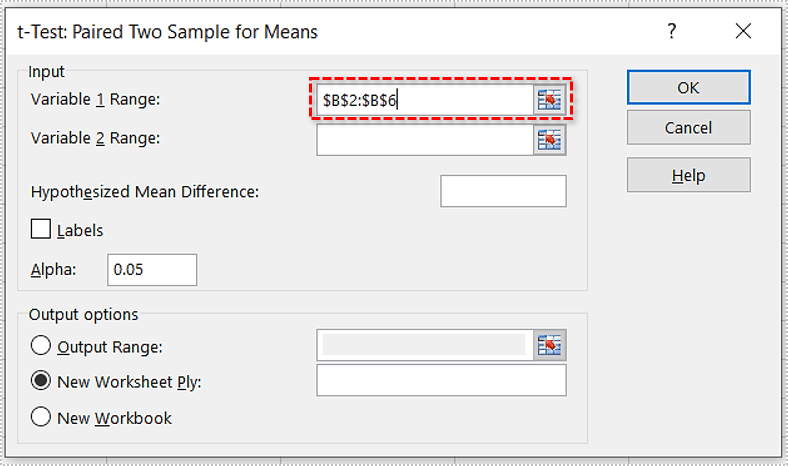8. Enter the second range/argument. In this case, it is C2:C6.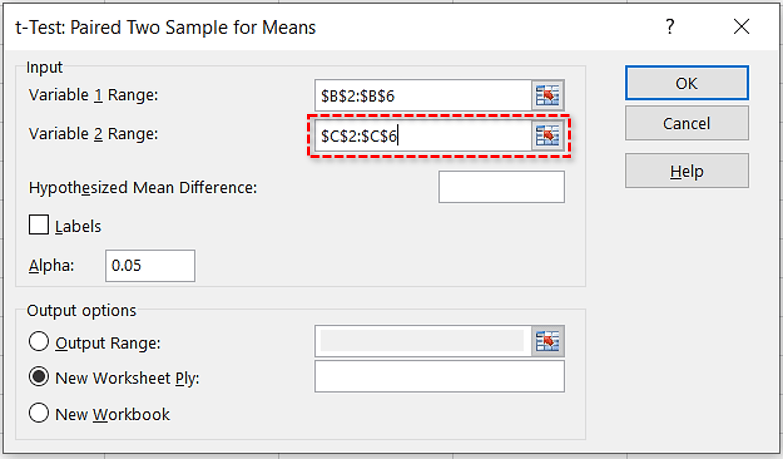9. Leave the default value in the Alpha text box (it’s 0.05).10. Click on the Output Range radio button and pick where you want the result. If it’s the A8 cell, type in: \$A\$8.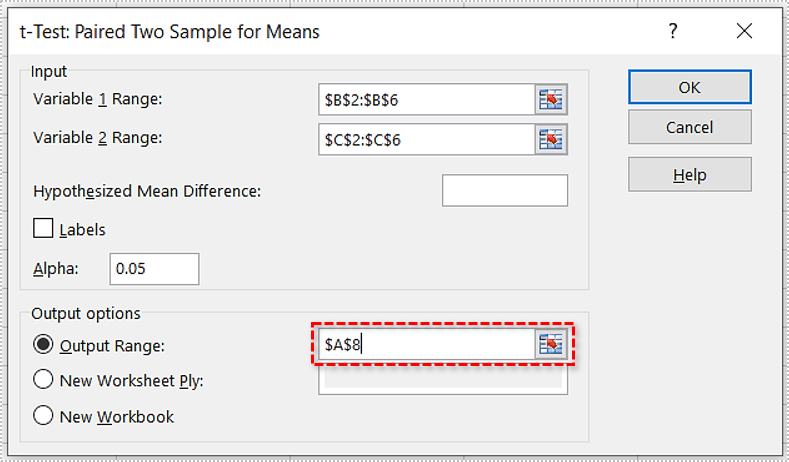11. Click OK.12. Excel will calculate the p-value and several other parameters. The final table might look like this:As you can see, the one-tail p-value is the same as in the first case – 0.133905569. Since it is above 0.05, the null hypothesis applies for this table, and the evidence against it is weak.

## Finding the p-Value in Excel 2016

Similar to the steps above, let’s cover calculating the p-Value in Excel 2016.

1. We’ll be using the same example as above, so create the table if you want to follow along.2. Now, in Cell A8, type =T.Test(B2:B6, C2:C6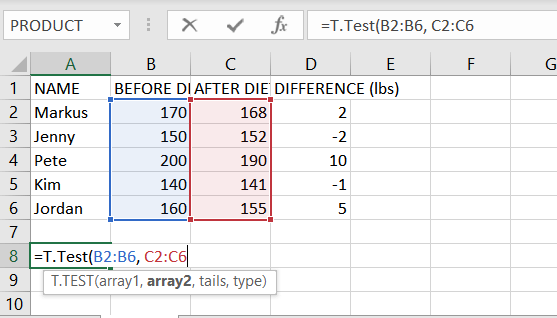3. Next, in Cell A8, enter a comma after C6 and then select One-tailed distribution.

4. Then, enter another comma and select Paired.

5. The equation should now be =T.Test(B2:B6, C2:C6,1,1).6. Finally, press Enter to show the result.The results may vary by a few decimal places depending upon your settings and available screen space.

## Things to Know About the p-Value

Here are some useful tips regarding p-value calculations in Excel.

1. If the p-value is equal to 0.05 (5%), the data in your table is significant. If it is less than 0.05 (5%), the data you have is highly significant.

2. In case the p-value is more than 0.1 (10%), the data in your table is insignificant. If it’s in the 0.05-0.10 range, you have marginally significant data.

3. You can change the alpha value, though the most common options are 0.05 (5%) and 0.10 (10%).

4. Choosing two-tailed testing can be the better choice, depending on your hypothesis. In the example above, one-tailed testing means we explore whether the test subjects lost weight after dieting, and that is exactly what we needed to find out. But a two-tailed test would also examine whether they gained statistically significant amounts of weight.

5. The p-value can’t identify variables. In other words, if it identifies a correlation, it can’t identify the causes behind it.

## The p-Value Demystified

Every statistician worth his or her salt has to know the ins and outs of null hypothesis testing and what the p-value means. This knowledge will also come in handy to researchers in many other fields.

Have you ever used Excel to calculate the p-value of a statistical model? Which method did you use? Do you prefer another way to calculate it? Let us know in the comments section.